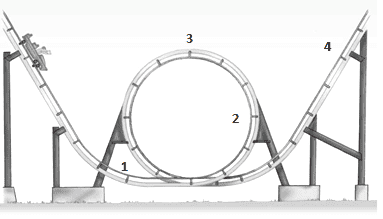# Rollercoaster velocity question, only given: radius of loop.

• MelissaJL
In summary, the rollercoaster moves freely with negligible friction and has a loop with a radius of 20m. At position 3, the car has a speed of 14m/s. Using conservation of energy, the speed at position 1 and 2 can be calculated to be 31.3m/s and 24.2m/s respectively. The difference in height between position 1 and 4 is 44.9m, with a speed of 10m/s at position 4. Mass is not needed for these calculations.

## Homework StatementThe rollercoaster moves freely without negligible friction. The radius of the loop is 20m and the car barely makes it through the loop.
Find the speed at position 3.
Find the speed at position 1 and 2.
Find the difference in height between position 1 and 4 if the speed at position 4 is 10m/s.

The only part I've been able to solve so far is position 3 which I used the formula:
v=√gR=√196=14m/s

I'm not sure what direction to take for position one and two and was wondering if I could get help with those two parts. Thank you so much :)

MelissaJL said:
... moves freely without negligible friction...

I think that you mean 'with negligible friction'.

For position 1 and 2 you can try using conservation of energy.

Oh yes. That is a typo, it moves with negligible friction. I understand that this is a conservation of energy but I am lost on how to use the formula without knowing the mass or height to then solve for velocity.

re speed at position 1:

Energy at 3 = energy at 1

What kind of energy at 3?
What kind of energy at 1?

Note that the height at 3 = 2 x radius and you do not need the mass because it eliminates itself.

Thanks I figured it out. The values are for point 1 the velocity is 31.3 m/s. For point 2 the velocity is 24.2m/s. Lastly, the height differences between 1 and 4 is 44.9m. That was great help. :)